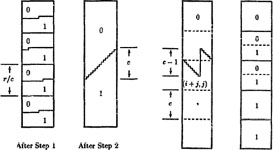﻿ Sorting Networks

# Sorting Networks

Sorting networks fascinate me. They even got me mentioned in Knuth Vol. 3, p. 666 of the second edition. My coauthor in this work is my Penn State PhD student Bruce Parker.

## The Pairwise Sorting Network (1992)Ian Parberry, "The Pairwise Sorting Network". Parallel Processing Letters, Vol. 2, No. 2,3, pp. 205-211, 1992. [pdf]

### Abstract

A new sorting network with exactly the same size and depth as the odd-even sorting network is presented. This sorting network is designed using the zero-one principle, and proceeds by first sorting pairs of bits and sorting the pairs into lexicographic order.

### Author's Comment

A 2010 paper by Michael Codish and Moshe Zazon-Ivry quotes my paper as saying: "It is the first sorting network to be competitive with the odd-even sort for all values of n. The value of the pairwise sorting network is not that it is superior to the odd-even sorting network in any sense, but that it is the first serious rival to appear in over 20 years", and they go on to say: "In this paper, almost 20 years after the introduction of pairwise sorting networks, we are in the position to state that pairwise sorting networks are significantly superior to odd-even sorting networks, at least for encoding cardinality constraints." Very cool.

## Sorting Network Verification Reloaded (1991)Ian Parberry, "On the Computational Complexity of Optimal Sorting Network Verification". Proceedings of The Conference on Parallel Architectures and Languages Europe, Springer-Verlag Lecture Notes in Computer Science, Vol. 506, pp. 252-269, June 1991. [pdf]

### Abstract

A sorting network is a combinational circuit for sorting, constructed from comparison-swap units. The depth of such a circuit is a measure of its running time. It is reasonable to hypothesize that only the fastest (that is, the shallowest) networks are likely to be fabricated. It is shown that the problem of verifying that a given sorting network actually sorts is Co-NP complete even for sorting networks of depth only $4 \log n + O(1)$ greater than optimal. This is shallower than previous depth bounds by a factor of two.

## Sorting Network Verification (1990)

Ian Parberry, "Single-Exception Sorting Networks and the Computational Complexity of Optimal Sorting Network Verification". Mathematical Systems Theory, Vol. 23, pp. 81-93, 1990.

### Abstract

A sorting network is a combinational circuit for sorting constructed from comparison-swap units. The depth of such a circuit is a measure of its running time. It is known that sorting-network verification is computationally intractable. However, it is reasonable to hypothesize that only the fastest (that is, the shallowest) networks are likely to be fabricated. It is shown that the verification of shallow sorting networks is also computationally intractable. Firstly, a method for constructing asymptotically optimal single-exception sorting networks is demonstrated. These are networks which sort all zero-one inputs except one. More specifically, their depth is $D(n-1)+2\log (n-1)+2$, where $D(n)$ is the minimum depth of an $n$-input sorting network. It follows that the verification problem for sorting networks of depth $2D(n) + 6 \log n + O(1)$ is Co-NP complete. Given the current state of knowledge about $D(n)$ for large $n$, this indicates that the complexity of verification for shallow sorting networks is as great as for deep networks

## 9-Input Sorting Networks (1989, 1991)

Ian Parberry, "A Computer Assisted Optimal Depth Lower Bound for Nine-Input Sorting Networks". Mathematical Systems Theory, Vol. 24, pp. 101-116, 1991. A preliminary version of this paper appeared in the Proceedings of Supercomputing '89, pp. 152-161, Reno, Nevada, Nov. 1989. [pdf]

### Abstract

It is demonstrated, using a combination of theoretical and experimental computer science, that there is no nine-input sorting network of depth six. If a nine-input sorting network of depth six exists, then there exists one with very special structure. There is an efficient algorithm for constructing and testing comparator networks of this form. This algorithm was implemented and executed on a supercomputer.

### Author's Comment

This paper had the good fortune to be mentioned on p. 666 of the second edition of Donald Knuth's The Art of Computer Programming, Vol. 3, Sorting and Searching.

## k-sorters (1989)Bruce Parker and Ian Parberry, "Constructing Sorting Networks from $k$-sorters", Information Processing Letters, Vol. 33, No. 3, pp. 157-162, 1989. [pdf]

### Abstract

We study the problem of using sorters of $k$ values to form large sorting and merging networks. For $n$ an integral power of $k$, we show how to merge $k$ sorted vectors of length $n/k$ each using $4 \log_k n - 3$ layers of $k$-sorters and $4 \log_k n - 5$ layers of k-input binary mergers. As a result, we show how to sort n values using $2 \log_k^2 n - \log_k n$ layers of $k$-sorters and $2 \log_k^2 n - 3 \log_k n + 1$ layers of $k$-input binary mergers.

Created April 21, 2010. Last updated August 23, 2019.